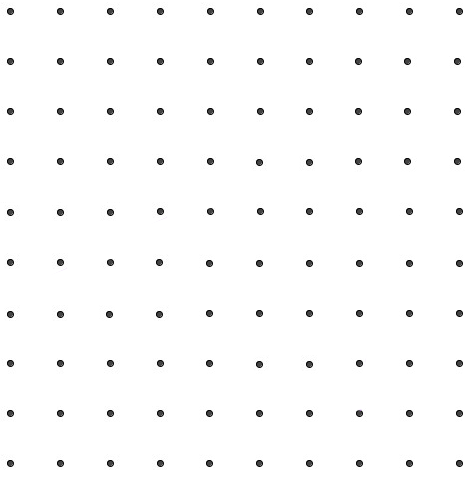# Finding Equilateral Triangles

Geometry Level 2Consider a $10 \times 10$ square grid of lattice points.

If we join the dots using straight lines, can we form an equilateral triangle?

×